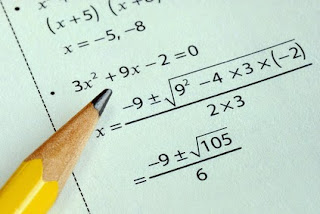# LET Reviewer in Math - Probability1. A coin is tossed 3 times. What is the probability of getting 2 heads and 1 tail?
a) 1/4
b) 3/8
c) 1/2
d) 5/8
e) none of the above

Solution: The total number of possible outcomes is 2^3 = 8 (since there are 2 options for each toss). There are 3 ways to get 2 heads and 1 tail: HHT, HTH, THH. Each of these outcomes has a probability of (1/2)^3 = 1/8. Therefore, the probability of getting 2 heads and 1 tail is:

3 x 1/8 = 3/8

2. A jar contains 5 red balls, 3 blue balls, and 2 green balls. If one ball is drawn at random, what is the probability of getting a blue ball?
a) 1/2
b) 1/4
c) 3/10
d) 3/4
e) none of the above

Solution: There are 10 balls in total, so the probability of getting a blue ball is 3/10.

3. A fair 6-sided die is rolled twice. What is the probability of getting a sum of 7?
a) 1/6
b) 1/5
c) 1/4
d) 1/3
e) none of the above

Solution: There are 36 possible outcomes when rolling two dice (since there are 6 options for each roll). There are 6 ways to get a sum of 7: (1,6), (2,5), (3,4), (4,3), (5,2), (6,1). Therefore, the probability of getting a sum of 7 is:

6/36 = 1/6

4. In a certain town, it is known that 30% of the people have a college degree. If 4 people from this town are randomly selected, what is the probability that all 4 have a college degree?
a) 0.001
b) 0.008
c) 0.027
d) 0.064
e) none of the above

Solution: The probability that any one person has a college degree is 0.3. Assuming independence between the people, the probability that all 4 have a college degree is:

0.3 x 0.3 x 0.3 x 0.3 = 0.0081

5. A bag contains 8 red balls and 4 blue balls. Two balls are drawn at random without replacement. What is the probability that both balls are red?
a) 1/3
b) 2/7
c) 2/3
d) 4/7
e) none of the above

Solution: The probability of drawing a red ball on the first draw is 8/12. If a red ball is drawn on the first draw, the probability of drawing another red ball on the second draw (without replacement) is 7/11. Therefore, the probability of drawing two red balls is:

(8/12) x (7/11) = 14/33

Answer: e) none of the given options.

6. A company produces light bulbs, and the probability of a bulb being defective is 0.05. A sample of 10 bulbs is chosen at random from the production line. What is the probability that at least 1 bulb is defective?
a) 0.401
b) 0.598
c) 0.950
d) 0.999
e) none of the above

Solution: The probability that none of the bulbs is defective is:

(0.95)^10 = 0.5987369392

Therefore, the probability that at least 1 bulb is defective is:

1 - 0.5987369392 = 0.4012630608

7. A class has 25 students, of which 10 are boys and 15 are girls. If 4 students are chosen at random, what is the probability that 2 are boys and 2 are girls?
a) 0.222
b) 0.329
c) 0.441
d) 0.556
e) none of the above

Solution: The number of ways to choose 2 boys out of 10 is C(10,2) = 45, and the number of ways to choose 2 girls out of 15 is C(15,2) = 105. The total number of ways to choose 4 students out of 25 is C(25,4) = 12,650. Therefore, the probability of choosing 2 boys and 2 girls is:

(45 x 105) / 12650 = 0.375

Answer: e) none of the given options.

8. In a certain town, it is known that 40% of the people have brown eyes and 60% have blue eyes. If 3 people from this town are randomly selected, what is the probability that all 3 have brown eyes?
a) 0.064
b) 0.096
c) 0.216
d) 0.343
e) none of the above

Solution: The probability that any one person has brown eyes is 0.4. Assuming independence between the people, the probability that all 3 have brown eyes is:

0.4 x 0.4 x 0.4 = 0.064

9. A bowl contains 5 red marbles and 3 green marbles. If 2 marbles are drawn at random without replacement, what is the probability that both marbles are red?
a) 1/14
b) 5/14
c) 5/21
d) 10/21
e) none of the above

Solution: The probability of drawing a red marble on the first draw is 5/8. If a red marble is drawn on the first draw, the probability of drawing another red marble on the second draw (without replacement) is 4/7. Therefore, the probability of drawing 2 red marbles is:

(5/8) x (4/7) = 5/14

10. A card is drawn at random from a standard deck of 52 playing cards. What is the probability that the card is a face card (jack, queen, or king) or a heart?
a) 13/52
b) 16/52
c) 20/52
d) 24/52
e) none of the above

Solution: There are 12 face cards in a deck of 52 playing cards, and there are 13 hearts. However, the jack, queen, and king of hearts are counted twice since they are both face cards and hearts. Therefore, the total number of cards that are either face cards or hearts is:

12 + 13 - 3 = 22

The probability of drawing a face card or a heart is therefore:

22/52 = 11/26

Answer: e) none of the given options.